A Survey of Gaussian Convolution Algorithms
FIR Gaussian convolution

Approximation by finite impulse response filtering. More...

## Detailed Description

Approximation by finite impulse response filtering.

This code approximates Gaussian convolution with finite impulse response (FIR) filtering. By truncating the Gaussian to a finite support, Gaussian convolution is approximated by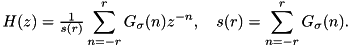The process to use these functions is the following:

1. fir_precomp() to precompute filter coefficients for the convolution
2. fir_gaussian_conv() or fir_gaussian_conv_image() to perform the convolution itself (may be called multiple times if desired)
3. fir_free() to clean up
Example

## Data Structures

struct  fir_coeffs_
Coefficients for FIR Gaussian approximation. More...

## Typedefs

typedef struct fir_coeffs_ fir_coeffs
Coefficients for FIR Gaussian approximation.

## Functions

static nummake_g_trunc (num sigma, long r)
Construct truncated Gaussian filter for FIR convolution. More...

static void conv_sym (num *dest, const num *src, long N, long stride, const num *h, long r)
Convolution with a symmetric filter. More...

int fir_precomp (fir_coeffs *c, double sigma, num tol)
Precompute filter coefficients for FIR filtering. More...

void fir_gaussian_conv (fir_coeffs c, num *dest, const num *src, long N, long stride)
FIR Gaussian convolution. More...

void fir_gaussian_conv_image (fir_coeffs c, num *dest, num *buffer, const num *src, int width, int height, int num_channels)
FIR filtering approximation of 2D Gaussian convolution. More...

void fir_free (fir_coeffs *c)
Release memory associated with fir_coeffs struct. More...

## Function Documentation

 static void conv_sym ( num * dest, const num * src, long N, long stride, const num * h, long r )
static

Convolution with a symmetric filter.

Parameters
 dest destination (must be distinct from src) src source signal N signal length stride stride between successive src and dest samples h symmetric filter, an array of length r + 1 r radius of filter h

This routine computes the convolution of src and h according to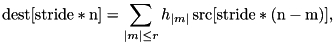where src is extrapolated with half-sample symmetry.

Definition at line 82 of file gaussian_conv_fir.c.

 void fir_free ( fir_coeffs * c )

Release memory associated with fir_coeffs struct.

Parameters
 c fir_coeffs created by fir_precomp()

Definition at line 197 of file gaussian_conv_fir.c.

 void fir_gaussian_conv ( fir_coeffs c, num * dest, const num * src, long N, long stride )

FIR Gaussian convolution.

Parameters
 c fir_coeffs created by fir_precomp() dest output convolved data (must be distinct from src) src data to be convolved N number of samples stride stride between successive samples

This routine approximates 1D Gaussian convolution with the FIR filter. The computation itself is performed by conv_sym().

Note
The computation is out-of-place, src and dest must be distinct.

Definition at line 137 of file gaussian_conv_fir.c.

 void fir_gaussian_conv_image ( fir_coeffs c, num * dest, num * buffer, const num * src, int width, int height, int num_channels )

FIR filtering approximation of 2D Gaussian convolution.

Parameters
 c fir_coeffs created by fir_precomp() dest destination image buffer array with at least width samples src source image, must be distinct from dest width image width height image height num_channels number of image channels

Similar to fir_gaussian_conv(), this routine performs 2D FIR-based Gaussian convolution.

Definition at line 158 of file gaussian_conv_fir.c.

 int fir_precomp ( fir_coeffs * c, double sigma, num tol )

Precompute filter coefficients for FIR filtering.

Parameters
 c fir_coeffs pointer to hold precomputed coefficients sigma Gaussian standard deviation tol filter accuracy (smaller tol implies larger filter)

This routine calls make_g_trunc() to construct the truncated Gaussian FIR filter. The radius of the filter is determined such that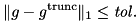By Young's inequality, the error between convolution using this truncated FIR filter and exact Gaussian convolution is bounded,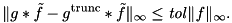Definition at line 117 of file gaussian_conv_fir.c.

 static num* make_g_trunc ( num sigma, long r )
static

Construct truncated Gaussian filter for FIR convolution.

Parameters
 sigma Gaussian standard deviation r radius of the filter
Returns
pointer to filter, or NULL on failure

This routine constructs a truncated Gaussian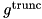for a specified radius r,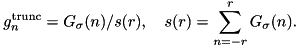The filter should be released by the caller with free().

Definition at line 43 of file gaussian_conv_fir.c.